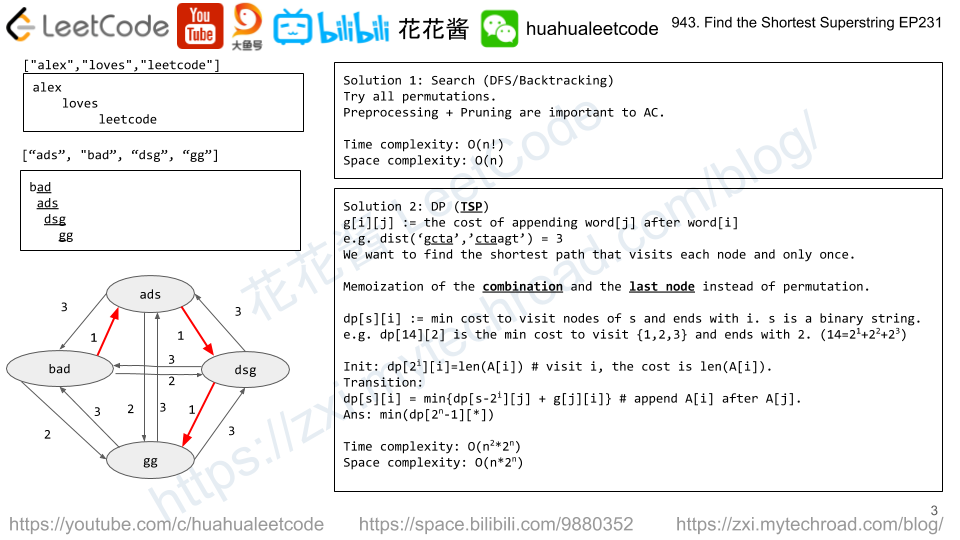Press "Enter" to skip to content

# Problem

You have an initial power P, an initial score of 0 points, and a bag of tokens.

Each token can be used at most once, has a value token[i], and has potentially two ways to use it.

• If we have at least token[i] power, we may play the token face up, losing token[i] power, and gaining 1 point.
• If we have at least 1 point, we may play the token face down, gaining token[i]power, and losing 1 point.

Return the largest number of points we can have after playing any number of tokens.

Example 1:

Input: tokens = , P = 50
Output: 0


Example 2:

Input: tokens = [100,200], P = 150
Output: 1


Example 3:

Input: tokens = [100,200,300,400], P = 200
Output: 2


Note:

1. tokens.length <= 1000
2. 0 <= tokens[i] < 10000
3. 0 <= P < 10000

# Solution: Greedy + Two Pointers

Sort the tokens, gain points from the low end gain power from the high end.

Time complexity: O(nlogn)

Space complexity: O(1)

# Problem

On a 2D plane, we place stones at some integer coordinate points.  Each coordinate point may have at most one stone.

Now, a move consists of removing a stone that shares a column or row with another stone on the grid.

What is the largest possible number of moves we can make?

Example 1:

Input: stones = [[0,0],[0,1],[1,0],[1,2],[2,1],[2,2]]
Output: 5


Example 2:

Input: stones = [[0,0],[0,2],[1,1],[2,0],[2,2]]
Output: 3


Example 3:

Input: stones = [[0,0]]
Output: 0


Note:

1. 1 <= stones.length <= 1000
2. 0 <= stones[i][j] < 10000

# Solution 2: Union Find

Find all connected components (islands)

Ans = # of stones – # of islands

# Problem

Given two sequences pushed and popped with distinct values, return true if and only if this could have been the result of a sequence of push and pop operations on an initially empty stack.

Example 1:

Input: pushed = [1,2,3,4,5], popped = [4,5,3,2,1]
Output: true
Explanation: We might do the following sequence:
push(1), push(2), push(3), push(4), pop() -> 4,
push(5), pop() -> 5, pop() -> 3, pop() -> 2, pop() -> 1


Example 2:

Input: pushed = [1,2,3,4,5], popped = [4,3,5,1,2]
Output: false
Explanation: 1 cannot be popped before 2.


Note:

1. 0 <= pushed.length == popped.length <= 1000
2. 0 <= pushed[i], popped[i] < 1000
3. pushed is a permutation of popped.
4. pushed and popped have distinct values.

# Solution: Simulation

Simulate the push/pop operation.

Push element from |pushed sequence| onto stack s one by one and pop when top of the stack s is equal the current element in the |popped sequence|.

Time complexity: O(n)

Space complexity: O(n)

# Problem

Given an array of integers A, a move consists of choosing any A[i], and incrementing it by 1.

Return the least number of moves to make every value in A unique.

Example 1:

Input: [1,2,2]
Output: 1
Explanation:  After 1 move, the array could be [1, 2, 3].


Example 2:

Input: [3,2,1,2,1,7]
Output: 6
Explanation:  After 6 moves, the array could be [3, 4, 1, 2, 5, 7].
It can be shown with 5 or less moves that it is impossible for the array to have all unique values.


Note:

1. 0 <= A.length <= 40000
2. 0 <= A[i] < 40000

# Solution: Greedy

Sort the elements, make sure A[i] >= A[i-1] + 1, if not increase A[i] to A[i – 1] + 1

Time complexity: O(nlogn)

Space complexity: O(1)

# Problem

Given an array A of strings, find any smallest string that contains each string in A as a substring.

We may assume that no string in A is substring of another string in A.

Example 1:

Input: ["alex","loves","leetcode"]
Output: "alexlovesleetcode"
Explanation: All permutations of "alex","loves","leetcode" would also be accepted.


Example 2:

Input: ["catg","ctaagt","gcta","ttca","atgcatc"]
Output: "gctaagttcatgcatc"

Note:

1. 1 <= A.length <= 12
2. 1 <= A[i].length <= 20# Solution 1: Search + Pruning

Try all permutations. Pre-process the cost from word[i] to word[j] and store it in g[i][j].

Time complexity: O(n!)

Space complexity: O(n)

# Solution 2: DP

g[i][j] is the cost of appending word[j] after word[i], or weight of edge[i][j].

We would like find the shortest path to visit each node from 0 to n – 1 once and only once this is called the Travelling sells man’s problem which is NP-Complete.

We can solve it with DP that uses exponential time.

dp[s][i] := min distance to visit nodes (represented as a binary state s) once and only once and the path ends with node i.

e.g. dp is the min distance to visit nodes (0, 1, 2) and ends with node 1, the possible paths could be (0, 2, 1), (2, 0, 1).

Time complexity: O(n^2 * 2^n)

Space complexity: O(n * 2^n)

## C++

Mission News Theme by Compete Themes.